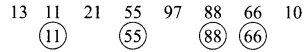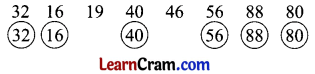# DAV Class 5 Maths Chapter 3 Worksheet 1 Solutions

The DAV Class 5 Maths Book Solutions Pdf and DAV Class 5 Maths Chapter 3 Worksheet 1 Solutions of Multiples and Factors offer comprehensive answers to textbook questions.

## DAV Class 5 Maths Ch 3 Worksheet 1 Solutions

Question 1.
Write the next four multiples of the first number in each case:
(a) 5, 10, 15, ____, ____, _____, _____
Solution:
5, 10, 15, 20, 25, 30, 35. (Recite the table of 5)

(b) 7, 14, 21, ____, ____, _____, _____
Solution:
7, 14, 21, 28, 35, 42, 49. (Recite the table of 7)

(c) 10, 20, 30, ____, ____, _____, _____
Solution:
10, 20, 30, 40, 50, 60, 70. (Recite the table of 10)

(d) 15, 30, 45, ____, ____, _____, _____
Solution:
15, 30, 45, 60, 75, 90, 105. (Recite the table of 15)

(e) 12, 24, 36, ____, ____, _____, _____
Solution:
12, 24, 36, 48, 60, 72, 84. (Recite the table of 12)Question 2.
Complete the following:
(a) 4th multiple of 5 is ____
Solution:
4th multiple of 5 is 20

(b) 5th multiple of 3 is ____
Solution:
5th multiple of 3 is 15

(c) 7th multiple of 8 is ____
Solution:
7th multiple of 8 is 56

(d) 6th multiple of 2 is ____
Solution:
6th multiple of 2 is 12

(e) 3rd multiple of 12 is ____
Solution:
3rd multiple of 12 is 36

Question 3.
Write the multiples of 6 between 20 and 40.
Solution:
24, 30, 36

Question 4.
Write the multiples of 7 which are less than 40.
Solution:
7, 14, 21, 28, 35Question 5.
Encircle the multiples of 11.
13  11  21  55  97  88  66  10
Solution:Question 6.
Encircle the multiples of 8.
32  16  19  40  46  56  88  80
Solution:Review Exercise

Question (i).
1 × 2 = 1 times 2 is 2
Solution:
1 × 2 = 1 times 2 is 2

(ii) 2 × 2 = ________
Solution:
2 × 2 = 2 times 2 is 4

(iii) 3 × 2 = ________
Solution:
3 × 2 = 3 times 2 is 6

(iv) 4 × 2 = ________
Solution:
4 × 2 = 4 times 2 is 8(v) 5 × 2 = ________
Solution:
5 × 2 = 5 times 2 is 10

(vi) 6 × 2 = ________
Solution:
6 × 2 = 6 times 2 is 12

(vii) 7 × 2 = ________
Solution:
7 × 2 = 7 times 2 is 14

(viii) 8 × 2 = ________
Solution:
8 × 2 = 8 times 2 is 16

(ix) 9 × 2 = ________
Solution:
9 × 2 = 9 times 2 is 18(x) 10 × 2 = ________
Solution:
10 × 2 = 10 times 2 is 20

DAV Class 5 Maths Chapter 3 Worksheet 1 Notes

• When a number divides another number exactly, then the dividend is called a multiple of the divisor.
e.g., 4 divides 32 exactly.
∴ 32 is a multiple of 4.
• When a number divides another number exactly then the divisor is called a factor.
e.g., 4 divides 12 exactly
∴ 4 is a factor of 12.
• Every number is multiple of itself.
• Every number is a multiple of 1.
• Numbers which are multiple of 2 are called even numbers.
• Numbers which are not multiples of 2 are called odd numbers.
• 1 is the common factor of any given number.
• A number that has only two factors is called a prime number.
• A number that has more than two factors is called a composite number.